# 1.1 Parametric equations  (Page 7/14)

 Page 7 / 14

## Key concepts

• Parametric equations provide a convenient way to describe a curve. A parameter can represent time or some other meaningful quantity.
• It is often possible to eliminate the parameter in a parameterized curve to obtain a function or relation describing that curve.
• There is always more than one way to parameterize a curve.
• Parametric equations can describe complicated curves that are difficult or perhaps impossible to describe using rectangular coordinates.

For the following exercises, sketch the curves below by eliminating the parameter t . Give the orientation of the curve.

$x={t}^{2}+2t,$ $y=t+1$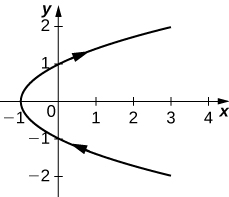orientation: bottom to top

$x=\text{cos}\left(t\right),y=\text{sin}\left(t\right),\left(0,2\pi \right]$

$x=2t+4,y=t-1$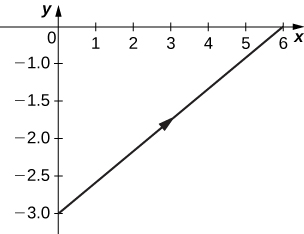orientation: left to right

$x=3-t,y=2t-3,1.5\le t\le 3$

For the following exercises, eliminate the parameter and sketch the graphs.

$x=2{t}^{2},\phantom{\rule{1em}{0ex}}y={t}^{4}+1$

$y=\frac{{x}^{2}}{4}+1$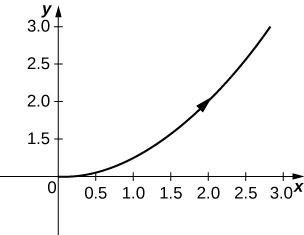For the following exercises, use technology (CAS or calculator) to sketch the parametric equations.

[T] $\begin{array}{cc}x={t}^{2}+t,\hfill & y={t}^{2}-1\hfill \end{array}$

[T] $\begin{array}{cc}x={e}^{\text{−}t},\hfill & y={e}^{2t}-1\hfill \end{array}$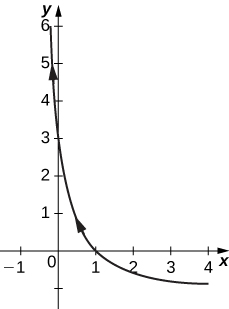[T] $\begin{array}{cc}x=3\phantom{\rule{0.2em}{0ex}}\text{cos}\phantom{\rule{0.2em}{0ex}}t,\hfill & y=4\phantom{\rule{0.2em}{0ex}}\text{sin}\phantom{\rule{0.2em}{0ex}}t\hfill \end{array}$

[T] $\begin{array}{cc}x=\text{sec}\phantom{\rule{0.2em}{0ex}}t,\hfill & y=\text{cos}\phantom{\rule{0.2em}{0ex}}t\hfill \end{array}$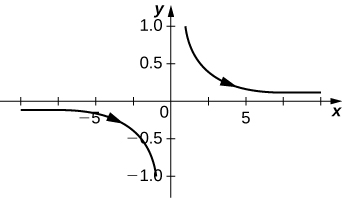For the following exercises, sketch the parametric equations by eliminating the parameter. Indicate any asymptotes of the graph.

$x={e}^{t},\phantom{\rule{1em}{0ex}}y={e}^{2t}+1$

$x=6\phantom{\rule{0.2em}{0ex}}\text{sin}\left(2\theta \right),y=4\phantom{\rule{0.2em}{0ex}}\text{cos}\left(2\theta \right)$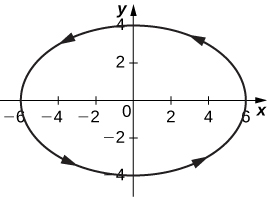$\begin{array}{cc}x=\text{cos}\phantom{\rule{0.2em}{0ex}}\theta ,\hfill & y=2\phantom{\rule{0.2em}{0ex}}\text{sin}\left(2\theta \right)\hfill \end{array}$

$\begin{array}{cc}x=3-2\phantom{\rule{0.2em}{0ex}}\text{cos}\phantom{\rule{0.2em}{0ex}}\theta ,\hfill & y=-5+3\phantom{\rule{0.2em}{0ex}}\text{sin}\phantom{\rule{0.2em}{0ex}}\theta \hfill \end{array}$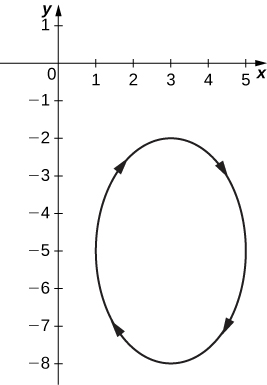$\begin{array}{cc}x=4+2\phantom{\rule{0.2em}{0ex}}\text{cos}\phantom{\rule{0.2em}{0ex}}\theta ,\hfill & y=-1+\text{sin}\phantom{\rule{0.2em}{0ex}}\theta \hfill \end{array}$

$\begin{array}{cc}x=\text{sec}\phantom{\rule{0.2em}{0ex}}t,\hfill & y=\text{tan}\phantom{\rule{0.2em}{0ex}}t\hfill \end{array}$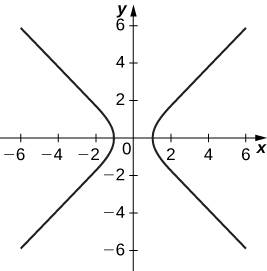Asymptotes are $y=x$ and $y=\text{−}x$

$\begin{array}{cc}x=\text{ln}\left(2t\right),\hfill & y={t}^{2}\hfill \end{array}$

$\begin{array}{cc}x={e}^{t},\hfill & y={e}^{2t}\hfill \end{array}$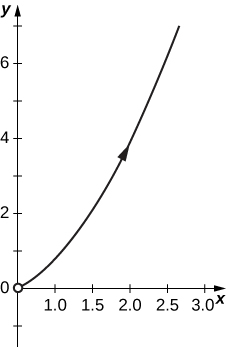$\begin{array}{cc}x={e}^{-2t},\hfill & y={e}^{3t}\hfill \end{array}$

$\begin{array}{cc}x={t}^{3},\hfill & y=3\phantom{\rule{0.2em}{0ex}}\text{ln}\phantom{\rule{0.2em}{0ex}}t\hfill \end{array}$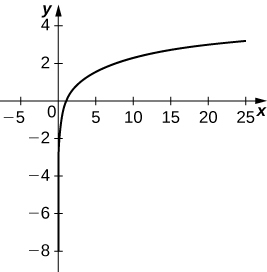$\begin{array}{cc}x=4\phantom{\rule{0.2em}{0ex}}\text{sec}\phantom{\rule{0.2em}{0ex}}\theta ,\hfill & y=3\phantom{\rule{0.2em}{0ex}}\text{tan}\phantom{\rule{0.2em}{0ex}}\theta \hfill \end{array}$

For the following exercises, convert the parametric equations of a curve into rectangular form. No sketch is necessary. State the domain of the rectangular form.

$\begin{array}{cc}x={t}^{2}-1,\hfill & y=\frac{t}{2}\hfill \end{array}$

$x=4{y}^{2}-1;$ domain: $x\in \left[1,\infty \right).$

$\begin{array}{cc}x=\frac{1}{\sqrt{t+1}},\hfill & y=\frac{t}{1+t},t>-1\hfill \end{array}$

$x=4\phantom{\rule{0.2em}{0ex}}\text{cos}\phantom{\rule{0.2em}{0ex}}\theta ,y=3\phantom{\rule{0.2em}{0ex}}\text{sin}\phantom{\rule{0.2em}{0ex}}\theta ,t\in \left(0,2\pi \right]$

$\frac{{x}^{2}}{16}+\frac{{y}^{2}}{9}=1;$ domain $x\in \left[-4,4\right].$

$\begin{array}{cc}x=\text{cosh}\phantom{\rule{0.2em}{0ex}}t,\hfill & y=\text{sinh}\phantom{\rule{0.2em}{0ex}}t\hfill \end{array}$

$\begin{array}{cc}x=2t-3,\hfill & y=6t-7\hfill \end{array}$

$y=3x+2;$ domain: all real numbers.

$\begin{array}{cc}x={t}^{2},\hfill & y={t}^{3}\hfill \end{array}$

$\begin{array}{cc}x=1+\text{cos}\phantom{\rule{0.2em}{0ex}}t,\hfill & y=3-\text{sin}\phantom{\rule{0.2em}{0ex}}t\hfill \end{array}$

${\left(x-1\right)}^{2}+{\left(y-3\right)}^{2}=1;$ domain: $x\in \left[0,2\right].$

$\begin{array}{cc}x=\sqrt{t},\hfill & y=2t+4\hfill \end{array}$

$\begin{array}{cc}x=\text{sec}\phantom{\rule{0.2em}{0ex}}t,\hfill & y=\text{tan}\phantom{\rule{0.2em}{0ex}}t,\pi \le t<\frac{3\pi }{2}\hfill \end{array}$

$y=\sqrt{{x}^{2}-1};$ domain: $x\in \left[-1,1\right].$

$\begin{array}{cc}x=2\phantom{\rule{0.2em}{0ex}}\text{cosh}\phantom{\rule{0.2em}{0ex}}t,\hfill & y=4\phantom{\rule{0.2em}{0ex}}\text{sinh}\phantom{\rule{0.2em}{0ex}}t\hfill \end{array}$

$\begin{array}{cc}x=\text{cos}\left(2t\right),\hfill & y=\text{sin}\phantom{\rule{0.2em}{0ex}}t\hfill \end{array}$

${y}^{2}=\frac{1-x}{2};$ domain: $x\in \left[2,\infty \right)\cup \left(\text{−}\infty ,-2\right].$

$x=4t+3,y=16{t}^{2}-9$

$\begin{array}{cc}x={t}^{2},\hfill & y=2\phantom{\rule{0.2em}{0ex}}\text{ln}\phantom{\rule{0.2em}{0ex}}t,t\ge 1\hfill \end{array}$

$y=\text{ln}\phantom{\rule{0.2em}{0ex}}x;$ domain: $x\in \left(0,\infty \right).$

$\begin{array}{cc}x={t}^{3},\hfill & y=3\phantom{\rule{0.2em}{0ex}}\text{ln}\phantom{\rule{0.2em}{0ex}}t,t\ge 1\hfill \end{array}$

$\begin{array}{cc}x={t}^{n},\hfill & y=n\phantom{\rule{0.2em}{0ex}}\text{ln}\phantom{\rule{0.2em}{0ex}}t,t\ge 1,\hfill \end{array}$ where n is a natural number

$y=\text{ln}\phantom{\rule{0.2em}{0ex}}x;$ domain: $x\in \left(0,\infty \right).$

$\begin{array}{c}x=\text{ln}\left(5t\right)\hfill \\ y=\text{ln}\left({t}^{2}\right)\hfill \end{array}$ where $1\le t\le e$

$\begin{array}{c}x=2\phantom{\rule{0.2em}{0ex}}\text{sin}\left(8t\right)\hfill \\ y=2\phantom{\rule{0.2em}{0ex}}\text{cos}\left(8t\right)\hfill \end{array}$

${x}^{2}+{y}^{2}=4;$ domain: $x\in \left[-2,2\right].$

$\begin{array}{c}x=\text{tan}\phantom{\rule{0.2em}{0ex}}t\hfill \\ y={\text{sec}}^{2}t-1\hfill \end{array}$

For the following exercises, the pairs of parametric equations represent lines, parabolas, circles, ellipses, or hyperbolas. Name the type of basic curve that each pair of equations represents.

$\begin{array}{c}x=3t+4\hfill \\ y=5t-2\hfill \end{array}$

line

$\begin{array}{c}x-4=5t\hfill \\ y+2=t\hfill \end{array}$

$\begin{array}{c}x=2t+1\hfill \\ y={t}^{2}-3\hfill \end{array}$

parabola

$\begin{array}{c}x=3\phantom{\rule{0.2em}{0ex}}\text{cos}\phantom{\rule{0.2em}{0ex}}t\hfill \\ y=3\phantom{\rule{0.2em}{0ex}}\text{sin}\phantom{\rule{0.2em}{0ex}}t\hfill \end{array}$

$\begin{array}{c}x=2\phantom{\rule{0.2em}{0ex}}\text{cos}\left(3t\right)\hfill \\ y=2\phantom{\rule{0.2em}{0ex}}\text{sin}\left(3t\right)\hfill \end{array}$

circle

$\begin{array}{c}x=\text{cosh}\phantom{\rule{0.2em}{0ex}}t\hfill \\ y=\text{sinh}\phantom{\rule{0.2em}{0ex}}t\hfill \end{array}$

$\begin{array}{c}x=3\phantom{\rule{0.2em}{0ex}}\text{cos}\phantom{\rule{0.2em}{0ex}}t\hfill \\ y=4\phantom{\rule{0.2em}{0ex}}\text{sin}\phantom{\rule{0.2em}{0ex}}t\hfill \end{array}$

ellipse

$\begin{array}{c}x=2\phantom{\rule{0.2em}{0ex}}\text{cos}\left(3t\right)\hfill \\ y=5\phantom{\rule{0.2em}{0ex}}\text{sin}\left(3t\right)\hfill \end{array}$

$\begin{array}{c}x=3\phantom{\rule{0.2em}{0ex}}\text{cosh}\left(4t\right)\hfill \\ y=4\phantom{\rule{0.2em}{0ex}}\text{sinh}\left(4t\right)\hfill \end{array}$

hyperbola

$\begin{array}{c}x=2\phantom{\rule{0.2em}{0ex}}\text{cosh}\phantom{\rule{0.2em}{0ex}}t\hfill \\ y=2\phantom{\rule{0.2em}{0ex}}\text{sinh}\phantom{\rule{0.2em}{0ex}}t\hfill \end{array}$

Show that $\begin{array}{c}x=h+r\phantom{\rule{0.2em}{0ex}}\text{cos}\phantom{\rule{0.2em}{0ex}}\theta \hfill \\ y=k+r\phantom{\rule{0.2em}{0ex}}\text{sin}\phantom{\rule{0.2em}{0ex}}\theta \hfill \end{array}$ represents the equation of a circle.

Use the equations in the preceding problem to find a set of parametric equations for a circle whose radius is 5 and whose center is $\left(-2,\phantom{\rule{0.2em}{0ex}}3\right).$

For the following exercises, use a graphing utility to graph the curve represented by the parametric equations and identify the curve from its equation.

[T] $\begin{array}{c}x=\theta +\text{sin}\phantom{\rule{0.2em}{0ex}}\theta \hfill \\ y=1-\text{cos}\phantom{\rule{0.2em}{0ex}}\theta \hfill \end{array}$

The equations represent a cycloid.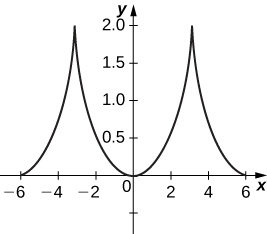[T] $\begin{array}{c}x=2t-2\phantom{\rule{0.2em}{0ex}}\text{sin}\phantom{\rule{0.2em}{0ex}}t\hfill \\ y=2-2\phantom{\rule{0.2em}{0ex}}\text{cos}\phantom{\rule{0.2em}{0ex}}t\hfill \end{array}$

[T] $\begin{array}{c}x=t-0.5\phantom{\rule{0.2em}{0ex}}\text{sin}\phantom{\rule{0.2em}{0ex}}t\hfill \\ y=1-1.5\phantom{\rule{0.2em}{0ex}}\text{cos}\phantom{\rule{0.2em}{0ex}}t\hfill \end{array}$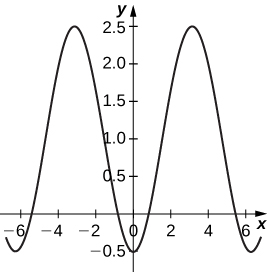An airplane traveling horizontally at 100 m/s over flat ground at an elevation of 4000 meters must drop an emergency package on a target on the ground. The trajectory of the package is given by $x=100t,y=-4.9{t}^{2}+4000,t\ge 0$ where the origin is the point on the ground directly beneath the plane at the moment of release. How many horizontal meters before the target should the package be released in order to hit the target?

The trajectory of a bullet is given by $x={v}_{0}\left(\text{cos}\phantom{\rule{0.2em}{0ex}}\alpha \right)\phantom{\rule{0.2em}{0ex}}ty={v}_{0}\left(\text{sin}\phantom{\rule{0.2em}{0ex}}\alpha \right)\phantom{\rule{0.2em}{0ex}}t-\frac{1}{2}g{t}^{2}$ where ${v}_{0}=500\phantom{\rule{0.2em}{0ex}}\text{m/s,}$ $g=9.8=9.8{\phantom{\rule{0.2em}{0ex}}\text{m/s}}^{2},$ and $\alpha =30\phantom{\rule{0.2em}{0ex}}\text{degrees}.$ When will the bullet hit the ground? How far from the gun will the bullet hit the ground?

22,092 meters at approximately 51 seconds.

[T] Use technology to sketch the curve represented by $x=\text{sin}\left(4t\right),y=\text{sin}\left(3t\right),0\le t\le 2\pi .$

[T] Use technology to sketch $x=2\phantom{\rule{0.2em}{0ex}}\text{tan}\left(t\right),y=3\phantom{\rule{0.2em}{0ex}}\text{sec}\left(t\right),\text{−}\pi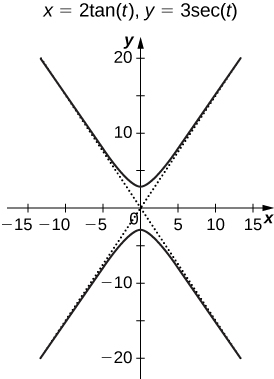Sketch the curve known as an epitrochoid , which gives the path of a point on a circle of radius b as it rolls on the outside of a circle of radius a . The equations are

$\begin{array}{}\\ \\ x=\left(a+b\right)\text{cos}\phantom{\rule{0.2em}{0ex}}t-c·\text{cos}\left[\frac{\left(a+b\right)t}{b}\right]\hfill \\ y=\left(a+b\right)\text{sin}\phantom{\rule{0.2em}{0ex}}t-c·\text{sin}\left[\frac{\left(a+b\right)t}{b}\right].\hfill \end{array}$
Let $a=1,b=2,c=1.$

[T] Use technology to sketch the spiral curve given by $x=t\phantom{\rule{0.2em}{0ex}}\text{cos}\left(t\right),y=t\phantom{\rule{0.2em}{0ex}}\text{sin}\left(t\right)$ from $-2\pi \le t\le 2\pi .$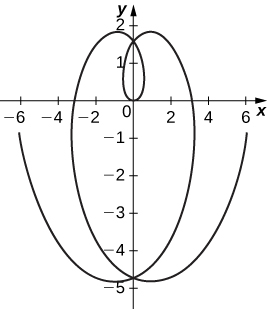[T] Use technology to graph the curve given by the parametric equations $x=2\phantom{\rule{0.2em}{0ex}}\text{cot}\left(t\right),y=1-\text{cos}\left(2t\right),\text{−}\pi \text{/}2\le t\le \pi \text{/}2.$ This curve is known as the witch of Agnesi.

[T] Sketch the curve given by parametric equations $\begin{array}{c}x=\text{cosh}\left(t\right)\hfill \\ y=\text{sinh}\left(t\right),\hfill \end{array}$ where $-2\le t\le 2.$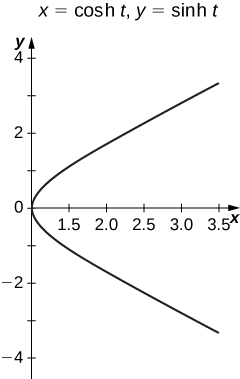where we get a research paper on Nano chemistry....?
nanopartical of organic/inorganic / physical chemistry , pdf / thesis / review
Ali
what are the products of Nano chemistry?
There are lots of products of nano chemistry... Like nano coatings.....carbon fiber.. And lots of others..
learn
Even nanotechnology is pretty much all about chemistry... Its the chemistry on quantum or atomic level
learn
da
no nanotechnology is also a part of physics and maths it requires angle formulas and some pressure regarding concepts
Bhagvanji
hey
Giriraj
Preparation and Applications of Nanomaterial for Drug Delivery
revolt
da
Application of nanotechnology in medicine
what is variations in raman spectra for nanomaterials
ya I also want to know the raman spectra
Bhagvanji
I only see partial conversation and what's the question here!
what about nanotechnology for water purification
please someone correct me if I'm wrong but I think one can use nanoparticles, specially silver nanoparticles for water treatment.
Damian
yes that's correct
Professor
I think
Professor
Nasa has use it in the 60's, copper as water purification in the moon travel.
Alexandre
nanocopper obvius
Alexandre
what is the stm
is there industrial application of fullrenes. What is the method to prepare fullrene on large scale.?
Rafiq
industrial application...? mmm I think on the medical side as drug carrier, but you should go deeper on your research, I may be wrong
Damian
How we are making nano material?
what is a peer
What is meant by 'nano scale'?
What is STMs full form?
LITNING
scanning tunneling microscope
Sahil
how nano science is used for hydrophobicity
Santosh
Do u think that Graphene and Fullrene fiber can be used to make Air Plane body structure the lightest and strongest. Rafiq
Rafiq
what is differents between GO and RGO?
Mahi
what is simplest way to understand the applications of nano robots used to detect the cancer affected cell of human body.? How this robot is carried to required site of body cell.? what will be the carrier material and how can be detected that correct delivery of drug is done Rafiq
Rafiq
if virus is killing to make ARTIFICIAL DNA OF GRAPHENE FOR KILLED THE VIRUS .THIS IS OUR ASSUMPTION
Anam
analytical skills graphene is prepared to kill any type viruses .
Anam
Any one who tell me about Preparation and application of Nanomaterial for drug Delivery
Hafiz
what is Nano technology ?
write examples of Nano molecule?
Bob
The nanotechnology is as new science, to scale nanometric
brayan
nanotechnology is the study, desing, synthesis, manipulation and application of materials and functional systems through control of matter at nanoscale
Damian
Is there any normative that regulates the use of silver nanoparticles?
what king of growth are you checking .?
Renato
What fields keep nano created devices from performing or assimulating ? Magnetic fields ? Are do they assimilate ?
why we need to study biomolecules, molecular biology in nanotechnology?
?
Kyle
yes I'm doing my masters in nanotechnology, we are being studying all these domains as well..
why?
what school?
Kyle
biomolecules are e building blocks of every organics and inorganic materials.
Joe
can you provide the details of the parametric equations for the lines that defince doubly-ruled surfeces (huperbolids of one sheet and hyperbolic paraboloid). Can you explain each of the variables in the equations?

#### Get Jobilize Job Search Mobile App in your pocket Now!By OpenStaxBy Cath YuBy OpenStaxBy John GabrieliBy Stephen VoronBy Brooke DelaneyBy OpenStaxBy OpenStaxBy Qqq QqqBy Janet Forrester